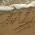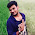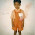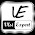## Index

 STA & SI Chapter1 Chapter2 Chapter3 Chapter4 Chapter5 Chapter6 Chapter7 Chapter8 Introduction Static Timing Analysis Clock Advance STA Signal Integrity EDA Tools Timing Models Other Topics

 Extraction & DFM Chapter1 Chapter2 Chapter3 Chapter4 Chapter5 Chapter6 Introduction Parasitic Interconnect Corner (RC Corner) Manufacturing Effects and Their Modeling Dielectric Layer Process Variation Other Topic

## Thursday, August 4, 2011

### "Delay - Timing path Delay" : Static Timing Analysis (STA) basic (Part 4a)

 STA & SI:: Chapter 2: Static Timing Analysis 2.1 2.2 2.3a 2.3b 2.3c 2.4a Timing Paths Time Borrowing Basic Concept Of Setup-Hold Basic Concept of Setup-Hold Violation Examples:S-H Time/Violation Timing Path Delay 2.4b 2.4c 2.5a 2.5b 2.6a 2.6b Interconnect Delay Models Delay - Wire Load Model Maximum Clock Frequency Calculate “Max Clock Freq”-Examples Fix Setup-Hold Violation-1 Fix Setup-Hold Violation-2 2.6c 2.7a 2.7b 2.7c 2.8 Fix Setup-Hold Violation-3 Incr/Decr Delay Method-1 Incr/Decr Delay Method-2 Incr/Decr Delay Method-3 10 ways to fix Setup-Hold Violation.

Static Timing analysis is divided into several parts:

This particular post is inspired by a question asked by Lalit. And Frankly speaking I am not able to resist myself to write a blog on this. I was thinking to capture all this since long but every time because of work  I have to drop my thoughts.. But today after reading his question.. I am not able to control myself. :)

So the Question is: (original question)

I have a doubt regarding how delay is calculated along a path.i think there are two ways
1) to calculate max delay and min delay, we keep adding max delays and min delays of all cells(buffer/inverter/mux) from start point to end point respectively.
2)in other way, we calculate path delay for rising edge and falling edge separately. we apply a rise edge at start point and keep adding cell delay. cell delay depends upon input transition and output fanout. so now we have two path delay values for rise edge and falling edge. greater one is considered as Max delay and smaller one is min delay.
which one is correct ?

Short Ans is .. both are correct and you have to use both. May be you all become confuse, so let me give you few details.

As I have mention that for Setup and Hold calculation , you have to calculate the Delay of the Timing path (capture path or launch path). Now in a circuit there are 2 major type of Delay.
1. CELL DELAY
• Timing Delay between an input pin and an output pin of a cell.
• Cell delay information is contained in the library of the cell. e.g- .lef file
2. NET DELAY.
• Interconnect delay between a driver pin and a load pin.
• To calculate the NET delay generally you require 3 most important information.
• Characteristics of the Driver cell (which is driving the particular net)
• Load characteristic of the receiver cell. (which is driven by the net)
• RC (resistance capacitance) value of the net. (It depends on several factor- which we will discuss later)
Both the delay can be calculated by multiple ways. It depends at what stage you require this information with in the design. e.g During pre layout or Post layout or during Signoff timing. As per the stage you are using this, you can use different ways to calculate these Delay. Sometime you require accurate numbers and sometime approximate numbers are also sufficient.

Now lets discuss this with previous background and then we will discuss few new concepts.

Now in the above fig- If I will ask you to calculate the delay of the circuit, then the delay will be

Delay=0.5+0.04+0.62+0.21+0.83+0.15+1.01+0.12+0.57=4.05ns (if all the delay in ns)

Now lets add few more value in this. As we know that every gate and net has max and min value, so in that case we can find out the max delay and min delay. (on what basis these max delay and min delay we are calculating .. we will discuss after that)

So in the above example, first value is max value and 2nd value is min value. So

Delay(max)= 0.5+0.04+0.62+0.21+0.83+0.15+1.01+0.12+0.57=4.05ns
Delay(min)= 0.4+0.03+0.6+0.18+0.8+0.1+0.8+0.1+0.5=3.51ns

Till now every one know the concept. Now lets see what's the meaning of min and max delay.

The delay of a cell or net depends on various parameters. Few of them are listed below.
• Library setup time
• Library delay model
• External delay
• Cell drive characteristic
• Operating condition (PVT)
• Input skew
• Back annotated Delay
If any of these parameter vary , the delay vary accordingly. Few of them are mutually exclusive. and In that case we have to consider the effect of only one parameter at a time. If that's the case , then for STA, we calculated the delay in both the condition and then categorize them in worst (max delay) condition or the best condition (min delay). E.g- if a cell has different delay for rise edge and fall edge. Then we are sure that in delay calculation we have to use only one value. So as per their value , we can categorize fall and rise delay of all the cell in the max and min bucket. And finally we come up with max Delay and min delay.Information used in Cell and net delay calculation (Picture Source - Synopsys)
The way delay is calculated also depends which tool are you using for STA or delay calculation. Cadence may have different algorithm from Synopsys and same is the case of other vendor tools like mentor,magma and all. But in general the basic or say concepts always remain same.
I will explain about all these parameter in detail in next of few blogs, but right now just one example which can help you to understand the situation when you have a lot of information about the circuit and you want to calculate the delay.

In the above diagram, you have 2 paths between UFF1 and UFF3. So when ever you are doing setup and hold analysis, these path will be the part of launch path (arrival time). So lets assume you want to calculate the max and min value of delay between UFF1 and UFF2.

Information1:
 UOR4 UNAND6 UNAND0 UBUF2 UOR2 DELAY(ns) 5 6 6 2 5

Calculation:
Delay in Path1 : 5+6=11ns,
Delay in Path2:  6+2+5+6=19ns,
So
Max Delay = 19ns - Path2 - Longest Path - Worst Path
Min Delay = 11ns - Path1 - Smallest Path - Best Path

Information2:
 UOR4 UNAND6 UNAND0 UBUF2 UOR2 Rise Delay (ns) 5 6 4 1 1 Fall Delay (ns) 6 7 3 1 1

Calculation:
Delay in Path1 :        Rise Delay : 5+6=11ns,              Fall Delay: 6+7=13ns
Delay in Path2:         Rise Delay : 4+1+1+6=12ns,      Fall Delay: 3+1+1+7=12ns
So
Max Delay = 13ns -Path1 (Fall Delay)
Min Delay = 11ns - Path1 (Rise Delay)

Note: here there are lot of more concepts which can impact the delay calculation sequence, like unate. We are not considering all those right now. I will explain later.

Information3:
 Library Delay UOR4 UNAND6 UNAND0 UBUF2 UOR2 Min Rise Delay (ns) 5 6 4 1 1 Fall Delay (ns) 6 7 3 1 1 Max Rise Delay (ns) 5.5 6.5 4.5 1.5 1.5 Fall Delay (ns) 5.5 6.5 2.5 0.5 0.5

Calculation:
For Min Library:
Delay in Path1 :        Rise Delay : 5+6=11ns,              Fall Delay: 6+7=13ns
Delay in Path2:         Rise Delay : 4+1+1+6=12ns,      Fall Delay: 3+1+1+7=12ns
For Max Library:
Delay in Path1 :        Rise Delay : 5.5+6.5=12ns,              Fall Delay: 5.5+6.5=14ns
Delay in Path2:         Rise Delay : 4.5+1.5+1.5+6.5=14ns,      Fall Delay: 2.5+0.5+0.5+6.5=10ns
So
Max Delay = 14ns- Path1(Fall Delay)/Path2(Rise Delay)
Min Delay = 10ns - Path2(Fall Delay)

As we have calculated above, STA tool also uses similar approach for finding the Max delay and Min Delay. Once Max and Min delay is calculated then during setup and hold calculation, we use corresponding value.

Once again I am mentioning that all these values are picked randomly. So it may be possible that practically the type/amount of variation in value is not possible.

In next part we will discuss these parameter in detail one by one.

1.Nice article. Many thanks to the author

2.Isn't propagating rise and fall delays without considering the transitions an over or under estimate as some transitions may not even be possible. Is there some way to workaround this without having to use the input vectors.

3.You are right but usually we do the worst case analysis. Or you can sat most of the STA tool do the calculation based like this. But if you need to check the actual calculation, then there is a methodology - know as PATH BASED ANALYSIS. In that we do delay calcualtion with respect to a particular path (timing path- please cehck the detials of timing path in my previous blogs.) And in that you can avoide all those transitions which is not possible.

4.one correction pls....When you explained Cell Delay you said it is mentioned in .lef file but it is in .lib file right?

5.This is the best explanation ever for Setup and hold timing and its violations.....I am waiting for your next blog....

1.thanks Maninder.
I was busy in last few months.. but soon you will see few more updates from my side.

6.Hi,
I have just started to understand about the STA concepts.
I got great idea about the setup and hold time from your blogs. Thank you for sharing this knowledge.
I want to know how can I apply these concepts in the real design. i.e If I want to add some constraints in the design then how can I add ?

1.In the real design - these concept help you to debug a lot of things and in understanding the reports and all ..
for applying the constraint - there is a file - SDC ( if you are using this - ), in the SDC, you can define the constraint as per your requirement.
I have written very introductory blog regarding the SDC file. please have a look in that.

http://vlsi-expert.blogspot.in/2011/02/synopsys-design-constraints-sdc-basics.html

I hope that will help you. In case you have any specific question , please let me know.

2.Thank you so much.
If I will face any difficulty then surely I will ask you.
Thanks again.

3.Respected Sir,

I am facing one error in SDC constraint.
Actually I have made one virtual clock for constrained Input and Output port.
As this clock is virtual, timing analyzer would not find any relationship between this clock and system clock.
But In my design It will give negative slack and defines relationship between system clock and virtual clock.
What would be the possible solution for this?

4.Hi,

Can you please let me know the commands and values /steps- you are using for constraining your input and output port?

Just one request (please dnt mind) - constraint related question- please ask in respective blog.It will help other people also.

5.It is ok. Next time I will ask questions related to the constraints in related blogs only.

7.This comment has been removed by the author.

8.Dear Sir, Really appreciate your handwork and sharing your knowledge to millions of ppl out there.

I just need to clear one doubt on calculating the longest path combinational delay: If a path consist of two gates U1 a nand and U2 a not with (rise, fall) timings as U1:(5,6), U2(8,4).Now(rise, fall) delays for the path are (5+8,6+4).As per your description above Max delay=13, min delay=10.
My doubt is, what if a 1->0 pulse is applied to inpiut of nand gate, whose other input is tied to 1? what should be the max dealy it encounters in reaching the output? it will be 6+8=14, and min dealy when a 0->1 transition at the i/p 5+4=9. Which is correct? Please clarify

9.Hi

I have a similar question like Sharan . If inverting logic is there one after another ,then how can you add all the rise delay ? Because at a time all the output cannot rise simultaneously.Is the approach mentioned by you is pessimistic ? If it is then how ?

1.hi,
whatever you/Sharan are saying is correct. But that's not the case when tool is calculating the delay. Because if that's the case then tool has to take care of all the combination and also the flow of the signal. Tool can do that but run time hit a lot in that case. So in all the EDA tool, there are ways/options to calculate the Delay. I can give you one very simple example -- like path based analysis and graph based analysis.

So in short, its like user can control the way .. how tool has to calculate the delay. But be more pessimistic , we just took the maximum of both the case(high to low and low to high) and calculate the worst(max)/best(min) delay.

I hope I have answered your questions. Please let me know if you have any further doubt here.

10.very nice work

11.Hi,
i have one doubt, is total delay of cell/net is sum of both rise and fall delay ?
in case yes,then why you made max/min delay as either rise or fall delay only?

12.Dear Sir,

All concepts are explained in such simple and elegant manner, even a beginner such as myself can grasp it quickly. Thanks a lot sharing your valuable knowledge and time with others.

Thanks!

13.Simply Superb ...well explained....

14.why we don't consider inductance in wire delay calculation??

1.We do that thing. But we have to understand the property of Inductance. For Inductance, rate of change of Current should be there. In digital design that's not the case.
So it's a design specific requirement.

2.Thank you so much for above answer

15.what actually is the difference between rise delay,fall delay and propagation delay???

16.Hi, thanks for your article. I have 1 small doubt. Isn't Path2 actually a false path?

1.Circuit is picked randomly. Path 2 can be or can't be False path. It depends on the other input of NOR and OR gate in path2.

17.Hi,
I didn't understand the max.delay equation written in information 1(max_delay=19ns-Path2-longest path-worst path). Can you explain it ?

18.Remarkable! Its genuinely amazing post, I have got much clear idea concerning from this article.

19.Can you explain the r and f written in path column of report.

20.Just want to say your article is as surprising. The clarity in your post is just cool and i can assume you're an expert on this subject.
Fine with your permission let me to grab your feed to keep
updated with forthcoming post. Thanks a million and please carry on the enjoyable work.